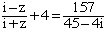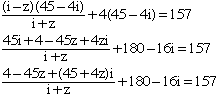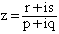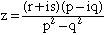Subject: Complex help needed Please can somebody help me. I am having enormous difficulty with one question in my maths homework. The question is shown below. If anybody out there can find the answers and show the workings and help me to understand. (a) Solve the equationwhere z is a complx number, expressing your answer in the form a + ib (b) Find the square root of 5 + 12i and hence the square root of -20 - 48i (c) Use the results of (b) to solve the quadratic equation z2 -(2 + 2i) z + (5 + 14i) = 0 Hence factorise the expression z2 -(2 + 2i) z + (5 + 14i) = 0 Hi Nick, For a I would first multiply both sides by 45 + 4i to getNow multiply through by i+z and collect all the terms containing z. The resulting expression will be of the form (p + iq)z = r + is and divide both sides by (p + iq) to getFinally multiply the numerator and denominator of the right side by (p - iq) to getand simplify. For b), if a + ib is a square root of 5 + 12i where a and b are real, then (a + ib)(a + ib) = 5 + 12i. That is a2 - b2 + 2abi = 5 + 12i Hence a2 - b2 = 5 and 2ab = 12 Solve these two equations for a and b. Cheers, Harley Go to Math Central